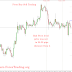## Top Ad unit 728 × 90

The Pivot buy Sell trading using pivot points to generate trading signals. Pivot points are a method of predicting support and resistance levels for the future.Using mathematical formulas the trader is able to know today, what will be the most powerful support and resistance levels tomorrow.
Don't worry, you don't need to calculate anything manually- there are indicators in any
trading platform that do that automatically.
If you are interested, here are the formula for Pivot calculation:
H – Yesterday's Highest Price
L – Yesterday's Lowest price
C – Yesterday's Close Price
P = (H + L + C) / 3.
R 1 = 2×P − L
S 1 = 2×P − H
R 2 = P + (H − L)
S 2 = P − (H − L)
R 3 = P + 2×(H − L)
S 3 = P − 2×(H − L)
Each of these points is a PIVOT POINT. The R points are Resistance levels that we will trade only with LONG trades, and the S points are Support level that we will trade only with SHORT trades.
Each day there are 5 pivot levels that we can trade. However we don't trade any pivot levels (it has to meet our filters).
First step is to confirm that the pivot level is strong.
We confirm that by demanding that price was in 30-25 pips distance from the pivot level at some point of the day. This makes sure that the pivot level is strong enough to trade:
After we confirm that the pivot level is good, we proceed by putting a trade order:
if the level is a RESISTANCE level we will enter a long trade when the pivot is broken, if the level is a SUPPORT level we will enter a short trade when the level is broken.
For long trades will put a buy order 1 pip above the level, and for short trades we will
put a sell order 1 pip below the order.
So if we have a Resistance pivot at 1.3119 the buy order will be placed at 1.3120
If we have a Support pivot at 1.3019 the sell order will be placed at 1.3018, and so on.

Stop Loss
We use a 20 pips trailing stop. This means that if price goes into +5 pips profit our stop loss will move 5 pips upwards, so the maximum price can go back before we exit the trade is 20 pips.

Take Profit
We exit half of the trade at +26 pips profit, and half the profit at +52 pips profit.
This is designed to get us in profit AND to take advantage of big moves.

Pivot Buy Sell TradingReviewed by learn forex trading on November 24, 2015 Rating: 5